# matplotlib.artist.Artist.set#

Artist.set(*, agg_filter=<UNSET>, alpha=<UNSET>, animated=<UNSET>, clip_box=<UNSET>, clip_on=<UNSET>, clip_path=<UNSET>, gid=<UNSET>, in_layout=<UNSET>, label=<UNSET>, mouseover=<UNSET>, path_effects=<UNSET>, picker=<UNSET>, rasterized=<UNSET>, sketch_params=<UNSET>, snap=<UNSET>, transform=<UNSET>, url=<UNSET>, visible=<UNSET>, zorder=<UNSET>)[source]#

Set multiple properties at once.

Supported properties are

Property

Description

`agg_filter`

a filter function, which takes a (m, n, 3) float array and a dpi value, and returns a (m, n, 3) array and two offsets from the bottom left corner of the image

`alpha`

scalar or None

`animated`

bool

`clip_box`

`Bbox`

`clip_on`

bool

`clip_path`

Patch or (Path, Transform) or None

`figure`

`Figure`

`gid`

str

`in_layout`

bool

`label`

object

`mouseover`

bool

`path_effects`

`AbstractPathEffect`

`picker`

None or bool or float or callable

`rasterized`

bool

`sketch_params`

(scale: float, length: float, randomness: float)

`snap`

bool or None

`transform`

`Transform`

`url`

str

`visible`

bool

`zorder`

float

## Examples using `matplotlib.artist.Artist.set`#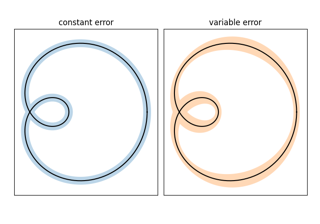Curve with error band

Curve with error band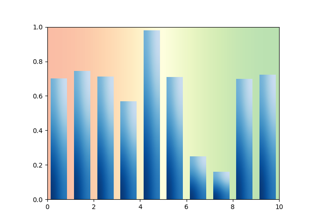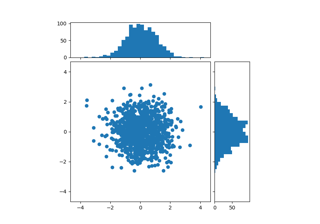Scatter plot with histograms

Scatter plot with histograms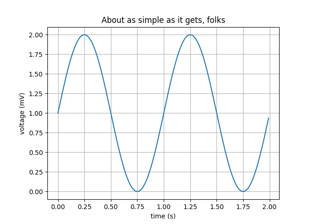Simple Plot

Simple Plot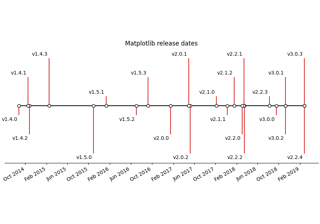Creating a timeline with lines, dates, and text

Creating a timeline with lines, dates, and text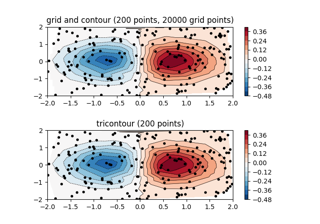Contour plot of irregularly spaced data

Contour plot of irregularly spaced data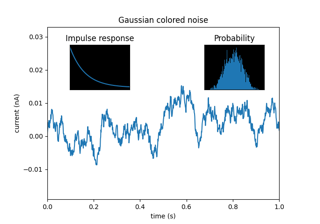Axes Demo

Axes Demo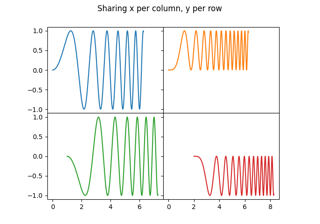Creating multiple subplots using plt.subplots

Creating multiple subplots using ``plt.subplots``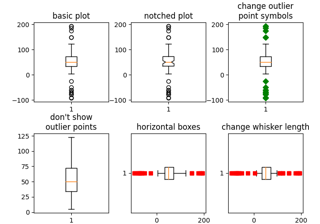Boxplots

Boxplots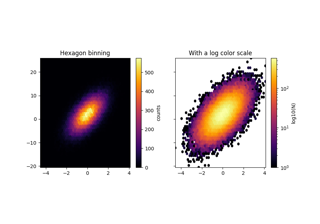Hexagonal binned plot

Hexagonal binned plot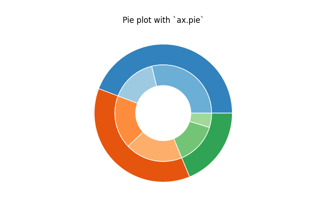Nested pie charts

Nested pie charts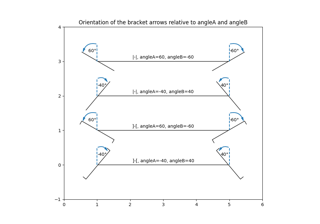Angle annotations on bracket arrows

Angle annotations on bracket arrows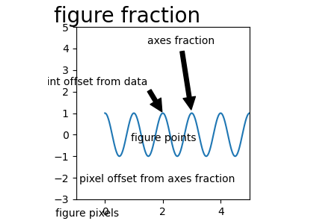Annotating Plots

Annotating Plots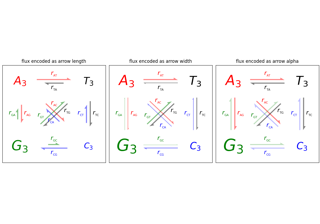Arrow Demo

Arrow Demo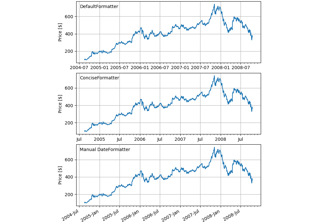Date tick labels

Date tick labels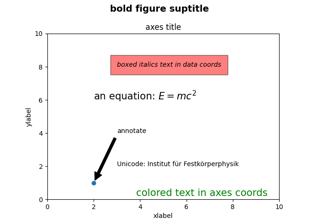Text Commands

Text Commands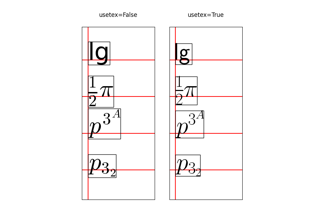Usetex Baseline Test

Usetex Baseline Test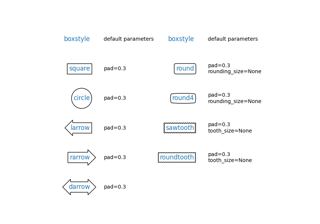Drawing fancy boxes

Drawing fancy boxes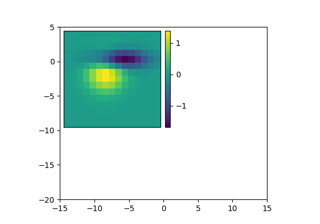Adding a colorbar to inset axes

Adding a colorbar to inset axes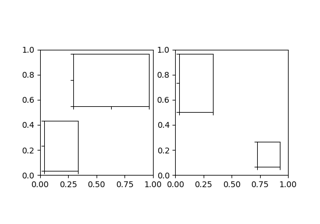Inset Locator Demo

Inset Locator Demo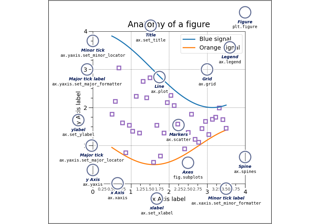Anatomy of a figure

Anatomy of a figureAnimated 3D random walk

Animated 3D random walkPick Event Demo

Pick Event Demo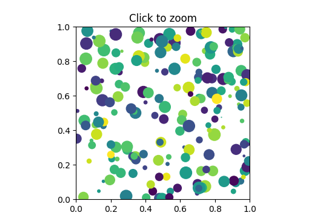Zoom Window

Zoom Window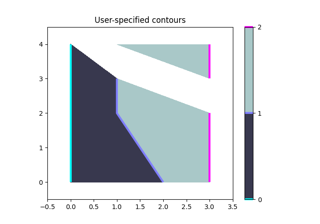Manual Contour

Manual Contour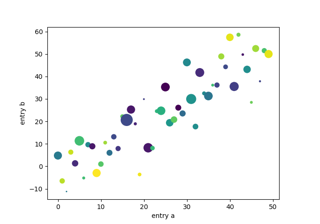Plotting with keywords

Plotting with keywords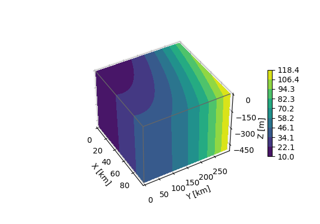3D box surface plot

3D box surface plot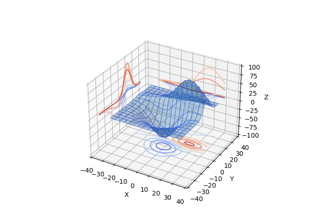Project contour profiles onto a graph

Project contour profiles onto a graph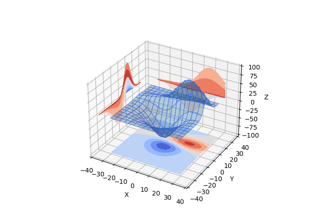Project filled contour onto a graph

Project filled contour onto a graph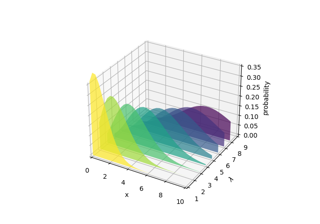Generate polygons to fill under 3D line graph

Generate polygons to fill under 3D line graph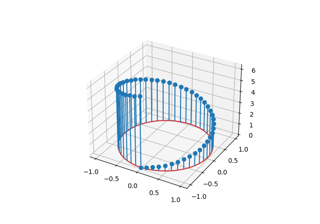3D stem

3D stem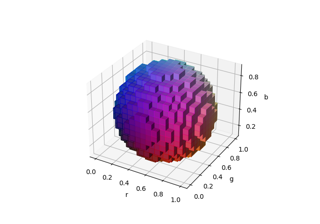3D voxel / volumetric plot with RGB colors

3D voxel / volumetric plot with RGB colors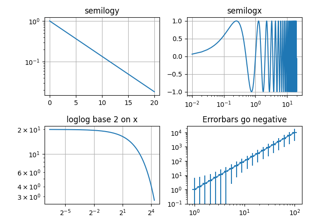Log Demo

Log Demo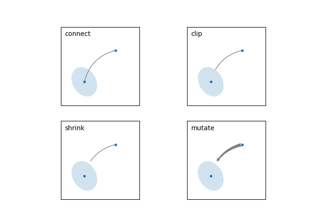Annotate Explain

Annotate Explain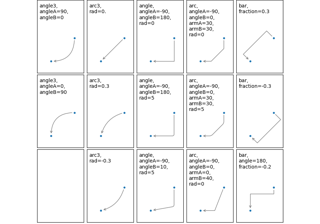Connection styles for annotations

Connection styles for annotations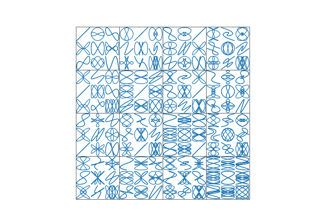Nested GridSpecs

Nested GridSpecs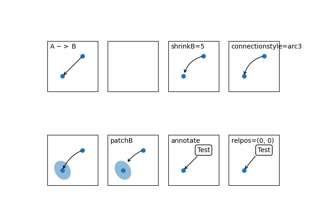Simple Annotate01

Simple Annotate01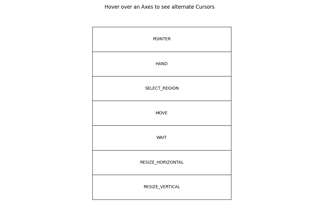Mouse Cursor

Mouse Cursor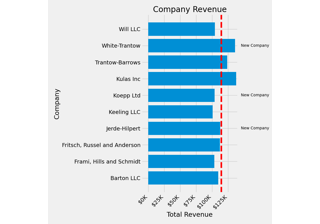The Lifecycle of a Plot

The Lifecycle of a Plot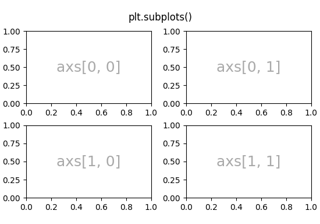Arranging multiple Axes in a Figure

Arranging multiple Axes in a Figure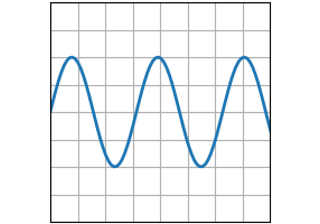plot(x, y)

plot(x, y)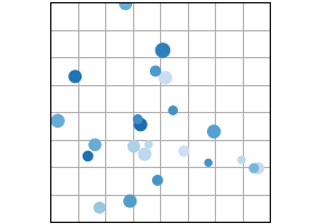scatter(x, y)

scatter(x, y)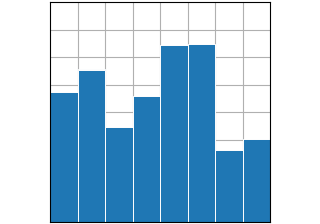bar(x, height)

bar(x, height)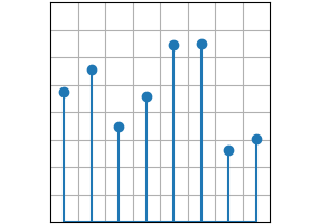stem(x, y)

stem(x, y)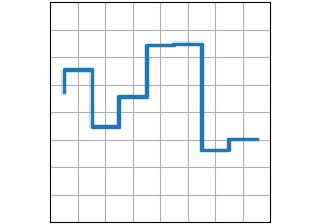step(x, y)

step(x, y)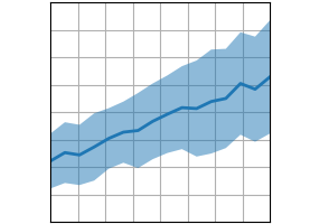fill_between(x, y1, y2)

fill_between(x, y1, y2)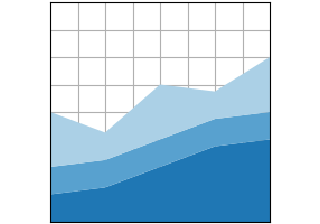stackplot(x, y)

stackplot(x, y)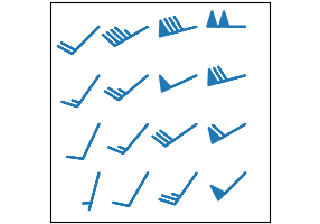barbs(X, Y, U, V)

barbs(X, Y, U, V)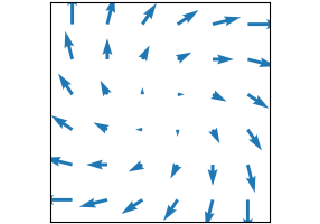quiver(X, Y, U, V)

quiver(X, Y, U, V)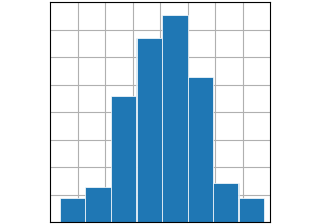hist(x)

hist(x)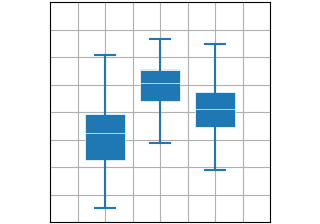boxplot(X)

boxplot(X)errorbar(x, y, yerr, xerr)

errorbar(x, y, yerr, xerr)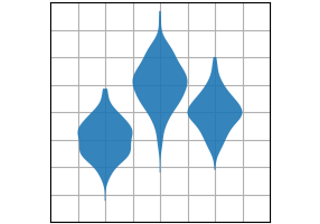violinplot(D)

violinplot(D)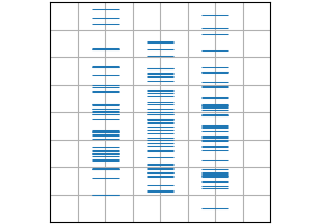eventplot(D)

eventplot(D)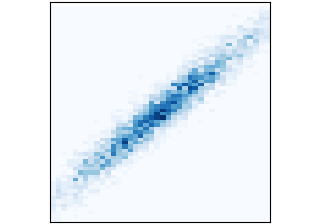hist2d(x, y)

hist2d(x, y)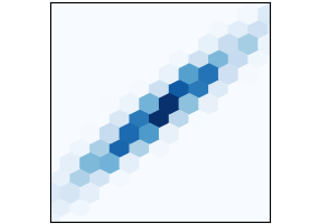hexbin(x, y, C)

hexbin(x, y, C)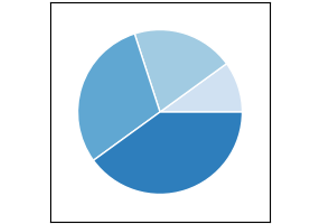pie(x)

pie(x)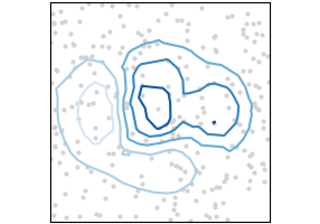tricontour(x, y, z)

tricontour(x, y, z)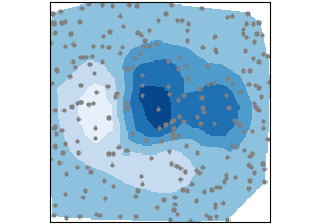tricontourf(x, y, z)

tricontourf(x, y, z)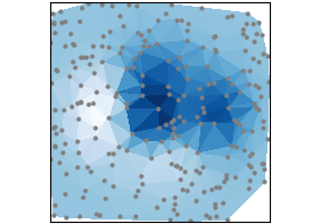tripcolor(x, y, z)

tripcolor(x, y, z)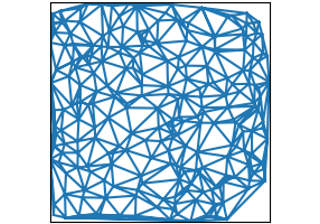triplot(x, y)

triplot(x, y)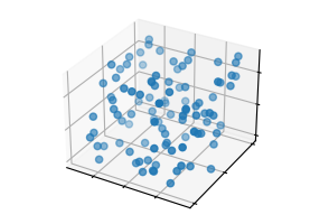3D scatterplot

3D scatterplot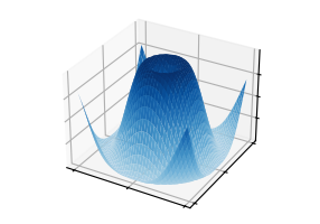3D surface

3D surface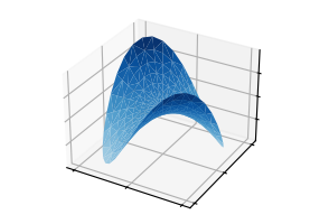Triangular 3D surfaces

Triangular 3D surfaces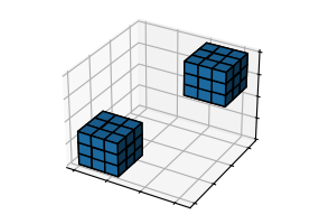3D voxel / volumetric plot

3D voxel / volumetric plot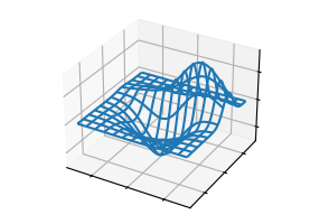3D wireframe plot

3D wireframe plot